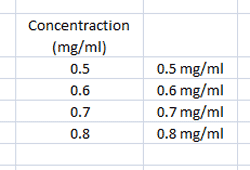# Excel Add Units to Cell

In Excel there is no direct method to add specialist units (mg/ml, km/h etc) to a numerical result contained in a cell. This tutorial will detail how to combine a numerical result with a specialist unit and make it appear in a single cell by using the =CellRef&" units" function.This tutorial will transform a numerical result (left) into a numerical result with text units (right)## Excel Add Units to Cell Tutorial

The =CellRef&" units" function combines the value in a give cell with the text combined between the quotation marks (" "). To add the units of mg/ml (concentration) to the value of 0.5 reported in cell B3 would be written as:

=B3&" mg/ml"

To add day units excel cell D5 which has a value of 10 would be entered as:

=D5&" days"

### Worked Example - Excel Add Units to Cell

This example will detail how to add units to a cell in excel. In the example there are four concentration values which have been entered into an excel spreadsheet. These values need to be exported into a Word document and with the measurements and units need to be in a single cell. The first stage is to hand enter the values into the spread sheet as shown below:Click in the cell where the value and units want to be displayed then in the function bar enter =CellRef&" units". In this worked example the value is =c4&" mg/ml" and will add (&) the text (between the " ") to the cell (C4)The cell now will display the value 0.5 mg/ml, please not this is now no longer a numerical value and units should only be added at the final stage of any calculation:As this is an excel (semi) function the information in the cell can be dragged and expanded using the usual excel features. The final stage is to drag the function down to include all of the values on the table.The finished table ready for exporting looks like this:Related Pages and Random Possibly Useful Guides